CALCULATING Lithology By Statistical Models

Determination of mineral rock composition is an important intermediate task of formation evaluation. In early days of well log analysis, only porosity, water saturation and sometimes permeability were calculated from the logs. Later on, significance of solid rock component distribution was recognized; while introduction of new well logging instruments enabled the more accurate assessment of lithology.

The goal of lithology interpretation is to divide the bulk rock volume into effective porosity and solid mineral components. The number of rock components involved in the analysis is dependent on the quality and quantity of available well logs. As a principle, the number of components can't exceed the number of input well logs plus one.

In a well of a carbonate reservoir where a rich set of well logs was measured, the following components may be determined:

•         Effective porosity
Calcite
Dolomite
Clay minerals (kaolinite, illite, chlorite etc.)
Silt
Ferroan minerals (oxides, hydroxides etc.).

In an older well of the same field with only a basic suite of well logs a three component model is applied:

•         Effective porosity
Carbonate (comprising calcite and dolomite)
Shale (comprising clays, silt, ferroan minerals).Rock Models

In typical circumstances, the number of rock components present in the formation exceeds the number which can be reliably determined. (It is typically not more than five, while in complex lithology the total number of applied mineral constituents may reach 15 or 20.)

In the case of a full log suite, the following models could be applied:

•         Porosity, calcite, dolomite, silt, kaolinite
Porosity, calcite, dolomite, silt, illite
Porosity, calcite, dolomite, kaolinite, Fe-minerals
Porosity, calcite, dolomite, illite, Fe-minerals.

It means that, for example, in a rock model either kaolinite or illite is present, but not both. It is a simplification, which is necessary to avoid unreliable solutions.Response Functions of Well Log Measurements

A great number of well logs are measured in recent wells. For the task of lithology determination, only those are involved which are sensitive for the mineral composition but are not sensitive for other conditions such as fluid saturations. The mathematical relationships connecting the rock composition to the well log measurements are called response functions.

Some typical examples:

Bulk density: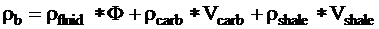Potassium content: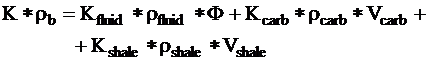Acoustic (Raymer equation):where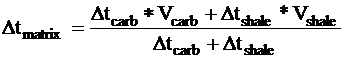Remarks:

(1)     Some well log measurements are related to the mass fractions of the rock components rather than to the volume fractions. In their response functions, volume fractions are multiplied by the specific density of the component. Further examples are: gamma ray, photoelectric effect, Thorium content.

(2)     Response functions may or may not be linear; example of the latter is the Raymer equation of acoustic sonic travel time. Similar is the case of SP (spontaneous potential). It means that the software should be prepared for the handling of systems of nonlinear equations.Zone Parameters

It can be observed in the examples of response functions that some parameters other than the volume fractions of minerals are involved. They are generally the specific values of the measured quantity for the rock component, e.g. the specific density. These are supposed to be known before the evaluation. They are called  "zone parameters" because their value is constant over a depth interval which covers (roughly) a geological formation.

Sources of information for zone parameters are the following:

In publications of well logging companies, handbooks etc. these parameters are published as glossary data; e.g. specific density of different minerals is well known from the literature.

The study of the measured well logs themselves (in the form of hard copies, crossplots etc.) can reveal some parameters. E.g. layers where shale content is near to 100 % can suggest the zone parameters of the "shale" rock component.

Log analysis can be calibrated to cores if they exist. In general, geological descriptions based on cores and drill cuttings reveal the minerals existing in the formation. Comparison of core porosity measurements with porosities computed from well logs may indicate that zone parameters of the "porosity logs" (density, neutron, sonic) are not correct.

In field-wide studies where several wells are measured in roughly the same period by the same logging company, experience from interpretation of one well can be transferred to another well regarding selection of rock components, rock models, zone parameters etc.

There is a feedback between zone parameters and the analysis. If there is a systematic difference between measured values and theoretical response values of a well log, it may be reduced by modifying zone parameters.Zones of ANALYSIs

Quantitative analysis of lithology in a well is carried out in a depth interval which is important regarding the hydrocarbon production; that is generally the potential reservoir interval and some adjacent intervals. It may be homogeneous in respect of lithology, but often it covers more than one geological formations which are distinctive in age, mineral composition etc. Rock components, rock models and zone parameters should be set up differently in these formations.

The basic zonation of the interpreted interval is controlled by the geological zonation in terms of rock formations.

Necessity of introducing different zones of interpretation may also emerge because of technical reasons. In some part of an interpreted formation quality and existence of well logging measurements may differ. For instance, some of the input well logs may be useless because of large rugosity effects. In that interval reduction of input information means reduction of the richness of outputs, e.g. less detailed rock models can be applied. This is done in the software by declaring the rugous interval as a different zone.

In the lithology part of the software, zones of interpretation are defined by listing the depth intervals belonging to that zone. For each rock component (mineral) and rock model, the list of zones where that particular rock component or model is applied should be declared. We can apply the same mineral in different zones with different zone parameters. In that case in output results the mineral can be displayed as the same in different zones, but during computation in each zone it is evaluated with its particular parameters in that zone.Deterministic analysis

In deterministic systems of interpretation the number of unknown volumetric fractions equals the number of equations (including the log response equations and the material balance equation). Validity of the result should be checked, e.g. the equations can yield negative volume fractions which should be avoided .

There are two approaches to this interpretation: sequential and simultaneous.

## Deterministic interpretation in a sequential way is the traditional (conventional) way of lithology determination. The volume fraction of one component is determined from one well log. (The response function of that well log is simplified so that only the volume fraction of that component is involved as the unknown quantity.) The second component is determined from another well log measurement; in its response function the volume fraction of the first component may be already involved. In a similar way at each step a new component is determined by using the response function of a well log measurement and volume fractions of components computed in previous steps. The last component is computed from the material balance equation.

A good example is determining the lithology of a shaly sandstone formation from gamma ray and neutron. In the first step, shale volume is computed from the formula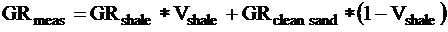In the second step (where Vshale is already known) porosity is computed from the neutron porosity measurement: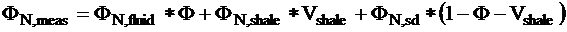In the last step the material balance equation yields the volume fraction of sand: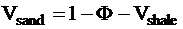Solving an actual interpretation task may be more complicated than this simple procedure. First, some constraints should applied, e.g. neither volume fraction can take negative values. Secondly, a branching can occur: different shale parameters can be applied if the points representing the depth sites separate into groups on a crossplot; in our case, on the crossplot of neutron porosity vs. gamma ray. (This corresponds to the application of different rock models in the statistical interpretation.)

## Deterministic interpretation by solving a system of equations is an alternate to sequential methods.

The response functions (plus the material balance equation) can be treated as a system of equations with the volume fractions of rock components as unknowns. This system of equations can be solved by appropriate mathematical methods. Advantages of this approach are:

All unknown volume fractions are computed simultaneously, so the complete forms of response functions are used (e.g. in the previous example effect of porosity on gamma ray measurement is not ignored).

Handling of constraints on the accepted range of volume fractions is more consistent. For example substituting zero values for negative volume fractions will lead us to more equations as unknowns so it leads us to the statistical interpretation.

Cumulative addition of errors associated to the sequential way is reduced.Statistical AnALYSIS

In the deterministic algorithms, the number of equations (with material balance) equals the number of unknown rock components. In statistical interpretation the number of equations exceeds the number of unknowns. It means that the number of well log measurements is at least as large as the number of rock components but generally larger. It means that the system is mathematically over-determined: no exact solution exists which satisfies all the equations.

The following method is applied for solving the task of statistical interpretation:

A measure of quality called incoherence is defined for the evaluation of each approximate solution for the system of equation;

A mathematical optimization problem is defined: find the set of volume fractions which gives the optimum value of the incoherence;

Constraints on the solution (upper and lower bounds on the volume fractions) are treated by including penalty terms in the quality indicator if the constraints are violated.

Advanced methods of mathematics are applied for the solution of the optimization problem; it yields a set of volume fractions of minerals as well as the value of incoherence.

The quality indicator is constructed by examining the reliability of each well log measurement involved. An error term is associated to each well log and the evaluation of each measurement by means of response function. The sources of error are the following:

Environmental effects: borehole enlargement and rugosity, interaction with drilling mud etc.;

Errors in depth matching and effects in difference of depth of investigation of the different well logging instruments;

Further on, the selection of rock models and zone parameters is burdened with errors.

All these diverse sources of error are added together and result in a random error for which we can assume a normal distribution with zero mean value and a standard deviation of si for the i-th measurement and answer.

The quality indicator, incoherence, is defined by the formula:where

bi:     measured value of the i-th well log;

bth,i: value computed from the response function of the i-th well log, called answer;

si:     standard deviation of error for the i-th measurement.

nf:    degree or number of freedom which equals:

number of well logs + 1 - number of unknowns

In a simple rock development, it is sufficient to apply a single rock model like the three-component sandstone model of porosity, sand (quartz) and shale. In real situations further minerals or other lithology components accumulate in the rock such as calcite, silt, clays, ferroan minerals etc. All of these components ought to be included in the interpretation, but their number would exceed the number of well log measurements. In that case multiple rock models are defined; in each of them the number of equations is greater than the number of components.

The statistical over-determined nature of the interpretation provides the quality indicator, incoherence, which enables us to select between the competing rock models. As a general principle, the rock model with the smallest incoherence is accepted as valid at each depth site. However, the software enables the overruling of this automatic model selection. The following reasons may verify the change of the least incoherence model:

The creation of longer homogeneous intervals requires the comparison of model selection for neighbouring depth sites and change of models if another model with only slightly larger incoherence fits better into the environment, according to the principle of geological consistency.

If the lithologic rock composition provided by the selected model contradicts our knowledge from other sources of information (e.g. cores), a more plausible model can be accepted.

Generally the interpretation is carried out in cycles: there is an initial selection of minerals, rock models and zone parameters. Then the interpretation is carried out and the results are examined. Occurrence of unexpectedly high incoherences indicates that the input of interpretation should be changed:

New minerals (rock components) should be included;

Further rock models should be applied;

Zone parameters should be modified.Statistical Method DETAILS

Well log measurements

Suppose L1, L2, ? Lm are well log measurements made in a borehole. A depth interval is selected where lithology should be evaluated: volumetric fractions of rock components (including porosity and solid minerals) should be determined. The interval is divided into zones (Z1, ?Zn) which are constructed of one or more sub-intervals. The Li log measurements are available at regular frequency - usually at each half foot.

The set of well log measurements involved in lithology determination can be changed in different zones. (E.g. gamma ray is involved in a shaly sandstone formation but it is abandoned in a shale-free carbonate zone.) It is assumed that all well log measurements are available in the zones where they are applied.

Rock components and models

The bulk rock volume is divided into effective porosity and solid rock components. The solid components may be minerals (calcite, quartz, kaolinite etc.) associations of minerals (ferroan minerals: oxides and hydroxides) or lithology types (limestone, sandstone, shale). Each rock component is characterized by its specific value of the applied rock measurements (zone parameters).

For each rock component its scope (i.e. the list of zones where it is applied) should be listed. A mineral can be present in one zone only or in several zones. In the latter case, its zone parameters may or may not be the same in different zones.

The number of mineral components present in a formation generally exceeds the number of rock components which can be reliably determined by the statistically over-determined interpretation technique.

Several subsets of rock components occurring together are defined and called rock models. For each model, the zones where it is applied should be listed.

Response functions and zone parameters

Response functions are mathematical relationships between the logging parameters and the rock mineral composition. These are theoretical functions which don't account for random errors or factors not involved in the model. Actual log measurements and theoretical responses generally differ.

The zone parameters are specific parameters of response functions which are constant for all depth sites in a zone of interpretation. Generally one zone parameter reflects the effect of a rock component in each response function.

System of equations

The set of response functions creates a system of equations together with the material balance equation; the latter describes the fact that the sum of all volume fractions in a unit volume equals one: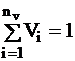It is included in the system of equations where it has a special status: while response functions are considered as approximations burdened with errors, the material balance equation is exact.

A system of equations is set up where the number of unknown rock volume fractions is smaller than the number of equations. The statistical over-determined nature of the method is characterized by the degree of freedom:

nf = nl + 1 - nv

Incoherence

Normalized incoherence is defined in Section 7. If the deviation of the measured logs and the theoretical responses is considered as a random error variable, the value of nf *.I2 has a chi-square distribution.

Normalized incoherence as measurement of quality of interpretation is used to the following purposes:

·          At each depth site with each model, the set of volume fractions which minimizes incoherence is accepted as the solution.

·          At each depth site where multiple models are applied, the model with the least incoherence is selected (if other considerations don't override it).

·          Statistical characteristics of incoherence in a well or in a zone are used as a general indicator of quality of interpretation. Improvement by changing selection of minerals, values of zone parameters, etc. is justified by the decrease of incoherence.Mathematical Solution

The mathematical problem that is to be solved for each model at each depth site is the following:

·          There is a system of equations consisting of nl + 1 equations and nv unknowns where nv < nl + 1 . The overdetermined nature of the system (more equations than unknowns) means that an exact mathematical solution generally is not found.

·          An object function (the normalized incoherence) is set up which describes the quality of each approximate solution of the system of equations. The goal is to find the set of volume fractions which minimizes the incoherence.

·          The restrictions on the valid range of volume fractions are taken into account. Penalty terms are added to the object function which assure that the final solution is inside the accepted range.

An iterative method is applied starting from an arbitrary initial approximation. A variant of the Newton method is applied which rapidly converges to the minimum place of the object function. (It is a local approximation of the function by a quadratic function at each step.)Presentation of Results

Strip logs of lithological composition are the usual way of graphical presentation of the interpretation. The volumetric fractions of the different rock components are displayed versus depth. At each depth site, the area covered by the specific colour code of the rock component is proportional to the volumetric fraction.

Statistical tables about abundances of rock components (total and separated by models) are printed together with the tables representing the rock models and zone parameters.

Although the strip logs of lithology are the most useful for the end user (geologist, reservoir engineer etc.), other forms of presentation are also important for the log analyst. The crossplots of response vs. measurement for the input well logs are crucial for the evaluation of the quality of interpretation. Clouds of points moved far away from the identity line may reflect two conditions:

The zone parameters of one (or more) minerals regarding that input log are not correct and should be modified;

Another rock component exists in the formation and it should be included in the rock models.

Crossplots of two rock components are suitable for investigating the reservoir quality of the formation. Especially, crossplot of volume fraction of shale vs. porosity is important in shaly sandstones. Irregularity of the crossplot (e.g. points with high porosity and high shale content) may refer to improper shale zone parameters.

Cumulative histogram of squared incoherence is used for the calibration of the standard errors of input logs so as to approximate the theoretical chi-square probability distribution.Optimization of Zone Parameters

Rock components involved in lithology interpretation are different in their degree of certainty. Some of them are stable minerals (such as quartz, calcite etc.) with well known attributes regarded as worldwide constants. Other components are more complex mixtures of minerals such as shale or ferroan minerals.
Their zone parameters are known only with a higher degree of uncertainty.

Another source of zone parameter uncertainty is the presence of systematic errors in well log measurements. Some of these errors can be eliminated by correction of the measured logs. However, often the deviation of zone parameters from their expected values is also necessary. In these cases adjustment of zone parameters is necessary. Beside manual improvement, necessity of automatic optimization emerges. The criterion of optimization is the magnitude of incoherence. Iterative methods can be applied by modifying the selected set of zone parameters until the minimum value of the weighted sum of squared incoherence is reached.

We have to emphasize that automatic zone parameter optimization should be applied with great care. Only one (or at most two) zone parameter of a rock component can be altered by this method; similarly, only a limited number of zone parameters belonging to the same log response function should be changed. Otherwise, artificial mathematical objects will be generated instead of real rock components.Role of Standard Errors

Another set of parameters which have great influence on lithology interpretation are the si standard errors associated with well logs included as input parameters. Both the magnitude of si-s relative to each other and their absolute value are important.

Increasing the value of a si term decreases the influence of the respective well log on the results of lithology interpretation. The crossplot of response vs. measurement of that log will show a wider cloud of points with statistical characteristics of regressional relationship degraded (the correlation coefficient decreases, closeness of regression coefficients to 1 and 0 decreases, error of estimation increases).

Decreasing the value of a si term increases the influence of the respective well log. The crossplot of response vs. measurement of that log will show a narrower cloud of points. The correlation coefficient increases, closeness of regression coefficients to 1 and 0 increases, error of estimation decreases.

In both cases, influence of other well logs on the interpretation changes the opposite way, e.g. increasing standard error of one log (i.e. decreasing influence of that log) will increase the influence of the other logs. One factor should be considered when evaluating the regressional relationship of response vs. measurement: the inherent variability of the measured well log values (reflecting the variability of the rock in respect of that well log) also affects the closeness of fit.

No fixed rules exist for the setting of relative magnitudes of standard errors Si, however, the following guidelines can be applied:

·          Ratios of standard errors are based on the ratios of measurement errors (repeat sections can be used for the evaluation of this).

·          The influence of the well logs should reflect their quality and their ability to reveal lithology in the formation. (E.g. gamma ray may have greater influence in shaly sandstones than in carbonates.)

·          Both extremities (input logs with negligible influence or with overwhelming influence) should be avoided.

The absolute values of standard errors si are calibrated by comparing the practical distribution of squared incoherences to the theoretical chi-square distribution. In FlexInLog, the upper quartile of the distribution is used as it is more robust parameter than the average. The si-s are multiplied with a common factor so that the upper quartile equals 0.5.In this way interpretations in different wells become comparable. E.g. depth sites where I2 > 2 considered as cases of high incoherence with unreliable lithology interpretation; calibration of standard errors assures that this criterion is consistent for different evaluations.Reducing Unknowns

Constraints on the valid range of rock components are applied in lithology determination. The trivial conditions of 0 £ Vi £ 1 should be met, besides, upper limits on some accessory minerals Vi £ (Vi)max << 1 may be applied. The most frequent case of violation is that the volume fraction of a component tends to be negative; the mathematical algorithm sets this volume fraction equal to zero.

Theoretically this means that the number of unknowns is reduced and the degree of freedom nf is increased by one. The increase of nf is applied in FlexInLog in the formula of the incoherence; this fact should be kept in mind when comparing incoherences of evaluations by models with different numbers of (existing) rock components.

A special case of this phenomenon occurs when deterministic evaluation is applied. Using a system of equation with nf = 0, an exact mathematical solution is computed. Generally, none of the constraints is violated and every equation is satisfied without error (responses equal measurements for each input well log).

If some constraints of avoiding negative rock components are violated, these components are substituted with zeroes. This reduces the number of unknown components and the system of equations is transformed into an over-determined one (nf > 0). The same mathematical algorithm can be used as for the statistical interpretation (standard errors should be defined). Responses are no longer equal to well log measurements, so incoherence can be computed and used for the assessment of the quality of interpretation.

The handling of violations of constraints this way provides some benefits of statistical interpretation for the deterministic interpretation. The mathematically optimal (least incoherence) solution is found if one or more of the volume fractions of rock components becomes zero.Determination of Matrix Density

Matrix density is obtained from the formula: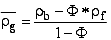where  rb: the measured (and corrected) log density

F : effective porosity provided by the quantitative lithological
interpretation

It is important to compare matrix density obtained from well log analysis with those measured on cores, since it can reveal some errors of lithological interpretation; for instance non-existing mineral or lithological components were taken into account in the applied rock models, what may cause serious difference between the two compared grain densities.

Page Views ---- Since 01 Jan 2015
Copyright 2023 by Accessible Petrophysics Ltd.
CPH Logo, "CPH", "CPH Gold Member", "CPH Platinum Member", "Crain's Rules", "Meta/Log", "Computer-Ready-Math", "Petro/Fusion Scripts" are Trademarks of the Author